Next: The Contradiction Backtracing Algorithm Up: COMP9417 Project Shapiro's Model Previous: COMP9417 Project Shapiro's Model

Subsections

# Introduction

This section is a brief introduction and overview of Machine Learning, Logic Programming, Inductive Logic Programming, and Shapiro's Model Inference System.

Source code for the applets used in this document can be found at: http://gungnir.csbnet.se/~peteg/thesis/src/prolog/prolog.html. (I've merged it with my thesis work).

## Logic Programming

Logic Programming is the area that broadly includes languages based in a fairly direct way on a logic, which is usually taken to be classical first-order Horn-clause logic. These semantical foundations are usually augmented with negation-as-finite-failure, meta-programming facilities (e.g. call/1, asserta/1, retract/1) and type, mode, and module systems. Prolog is the original such language, dating from the early 1970's, and lacks all such niceties except the first two.

A tutorial introduction to Prolog can be found in [CM81], and [Llo87b] provides a thorough treatment of the semantics of a purified version of the language.

In the following, we assume familiarity with logic programming. The specialised terminology of this field is described in Appendix A.

## Proof by Refutation

Prolog uses a form of resolution [Rob92] to execute logic programs. Restricted to Horn clauses, this proof technique uses the following rule of inference (a reworded version of [Sha81]):

Let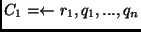(a definite query),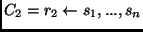(a definite clause) be sentences in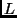. Provided that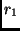and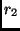have a most general unifier(i.e.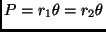), then the resolvent of these two clauses is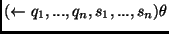, and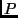is termed the atom resolved upon.is called the left component, and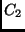the right component.
The aim is to derive the empty clause, written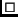, which is interpreted as contradiction''. This means that the query is inconsistent with the theory, from which we conclude that the negation of the query is in fact consistent with it. What's more, substitutions for the variables in the query that satisfy the latter are computed during the search for a refutation.

Horn clauses are particularly useful, as they have a very natural algorithmic interpretation; they have the flavour of the procedures found in imperative langauges. The output of such an executation is a sentence that satisfies the constraints asserted by the program, or failure if no such solution is found. In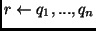,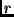is called the head of the clause and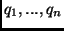the body. To execute the head, each conjunct of the body must be executed.

Refutation proof trees1 provide a way of visualising the logical aspect of executing a Prolog program, in contrast to the procedural (or operational) view that a debugger for an imperative language (such as C) would provide. The completed refutation tree should be read as because all the children are true, the parent is true in the context of the theory T''.

 Your browser does not support Java applets.

## Inductive Logic Programming

The goal of Inductive Logic Programming is to induce a compact, generalised, understandable-by-humans description of a set of facts in a very expressive formal language. The term was coined by Muggleton in 1991 [Sam93], but the field had been under active investigation for a long time before that. An overview of rule-learning (of which ILP is an instance) can be found in [Mit97, Chapter 10], and a substantial amount of ILP theory is presented in [NCdW97].

Given the gamut of programming languages, we might ask what it is about logic programming that makes it a good target for induction. We defer answering this question until we have presented the Model Inference System (Section 5) so that we have a point of reference.

Alternatively, it may be asked why a more expressive formal language such as full first-order logic is not employed. The main reason is efficiency: a theory induced by an ILP system is effectively and efficiently executable via a Prolog-like theorem prover, and does not require a massively non-deterministic search to determine whether a particular statement follows.

There is a plethora of ILP systems, e.g. FOIL [QCJ93], MARVIN [SB86], Progol [Mug95], and many variations on some core ideas. The two fundamental approaches taken to the induction problem are to exploit the properties of the description language (e.g. MIS) and to use a statistical model (e.g. FOIL). Any effective ILP system needs to combine them in some way: the former can greatly increase efficiency by better structuring the search space, and the latter is mandated by noisy (real-world) data. Again, we discuss these after presenting MIS.

In order to provide some intuition about ILP, the following points contrast it broadly with decision tree inducers [Mit97, Chapter 3 and 10]:

• Horn-clause logic is much more expressive than the attribute/value framework used by decision trees (which is effectively propositional, or zeroth-order, logic). This means that the task of inducing a theory is both much harder and much less efficient, and so ILP should only be used when this extra expressiveness is necessary.

More concretely, decision trees can only describe relationships between particular attributes and constants, whereas ILP can describe relationships amongst attributes.

• A theory induced by an ILP system can hold on an infinite domain; we might say the system can generalise to the infinite case. For example, MIS can learn an addition predicate that is defined on all triples of natural numbers.

For this to be the case, it must be possible to express recursive definitions in the hypothesis language2.

Indeed, as all finite relations can be encoded in a propositional language, this is at the core of ILP's power. A survey that focusses on precisely this topic is [FY99], where several techniques for providing structured recursion operators (such as divide-and-conquer) are discussed.

• ILP systems can (usually) make use of background knowledge, although it is a matter of current research as to how to do this efficiently.

## Philosophical Concerns

Abstractly, we might pose the question: just what is a valid inductive generalisation? From a classical philosophical viewpoint3, a satisfactory answer to this question is needed if we are ever to escape the I think, therefore I am'' solipsism of Descartes and be assured that it is possible to make valid assertions about the inter-subjective realm.

Hume [Hum85] argued quite forcefully in the eighteenth century that there can be no logically sound reason to generalise from experience to univerally true statements. The essence of his argument is that we must assume that nature is uniform if we were to grant validity to such statements, and that such an assumption cannot be verified indepedently of experience.

So, are we completely stuck? Surely not. In the context of machine learning we're simply looking for good descriptions that fit the data we have available - there is no inherent claim for universal generality here. Indeed, completeness is not always in itself desirable - a topic we take up in Section 3.

In contrast to empirical scepticism, we can simply assume that generalisations are necessary (perhaps as an aid to comprehending large amounts of data) and move along to the question of what a good way of discovering them is. In concert with this, we need to know what the requirements for effective learning are; can we, for example, learn simply by being exposed to positive examples of a concept?

While the former question is a matter of research, the latter one and others similar to it have been studied in some detail by computational learning theorists - see [JORS99] for an in-depth coverage of such.

Shapiro adopts the learning-in-the-limit model [Gol67] in order to prove various properties of his algorithms. Informally, the idea is that, given a presentation of facts in an observation language, the learner outputs a series of conjectures drawn from a hypothesis language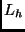that eventually converges to a finite description of them4. Note that there is no introspection requirement: the learner never realises that it has happened upon the correct description. Thus, the crux of Hume's argument - that we cannot establish uniformity - is side-stepped.

In the context of this model, both positive and negative examples are required; without negatives we could trivially output the everywhere-true program, and similarly for the no-positives case.

## An Overview of the Model Inference System

The Model Inference System, by Ehud Y. Shapiro [Sha81,Sha83] is an instance of the class of incremental generalise/specialise algorithms, which have the skeleton shown in Figure 1.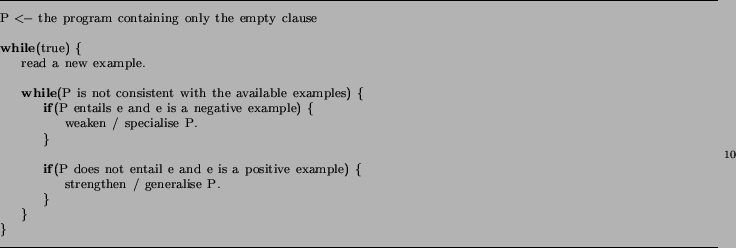Shapiro formalises the Model Inference Problem as follows [Sha81]:

Suppose we are given a first order languageand two subsets of it: an obervational languageand a hypothesis languagewith. In addition, assume that we are given an oracle for some unknown modelof. The Model Inference Problem is to find a finite-complete axiomatization of.
Unpacking this definition, we have the following:
• MIS takes as input a sequence of observations, where every statementin(eventually) appears and is flagged as either true or false. Such pairs are called facts.

• It is assumed that there is an oracle that can determine the truth of any ground statement inrelative to the model5. This kind of learning is termed supervised as it relies on the existence of an entity capable of answering questions about the domain, which is not always a reasonable assumption (Section 5).

The oracle can be formalised as a set facts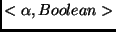, each of which reflect the truth or falsity ofin the target model.

• The output of this algorithm is a conjecture, which is a set of Horn clauses that entail all the positive observations, and none of the negatives, that have been presented to it so far.

The original MIS [Sha81] can actually learn theories in full first order logic, but we restrict it to Horn Clauses (i.e. Prolog rules) so that the learnt descriptions are executable. More technically, there are some restrictions on the languages involved; Shapiro proves a theorem that=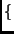ground atoms of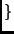,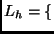Horn sentences ofis admissible, i.e. a model defined overis learnable in the limit.

MIS itself consists of two parts: roughly, a systematic way of finding bugs in programs (discussed in Section 2), and a search for fixes for these bugs (Section 3).

Both of these exploit the clean semantics of the pure Horn Clause core of Prolog, and hence run into difficulties with the non-logical parts of the language (e.g. the cut - !/0, unsound unification and the incompleteness of Prolog's proof strategy). Indeed, learning with the cut has since been shown to be difficult [BGT93].

Some further a priori observations:

• MIS assumes a fixed language; that is, it doesn't attempt to deal with the situation where a change in representation is required. In machine learning terms, it never tries to restrict the hypothesis language to the propositional sub-language of, even when the observations can be described in such a framework.

This is hardly surprising, however: it is quite difficult for even experts to invent effective transformations from real world'' domains to artificial languages.

• MIS is incremental, which means that a theory can always be later revised (although the state of the learner needs to be retained). This has implications for efficiency and completeness, which we discuss in more detail in Section 5.

• Shapiro proves a completeness result relative to Gold's learning in the limit framework with a most general refinement operator. Again, this is not of great practical interest for reasons detailed in Section 3.

• MIS can exploit background information, provided the system is made aware of it at the beginning of the induction process. See Section 5.1 for more discussion on background knowledge invention and use.Next: The Contradiction Backtracing Algorithm Up: COMP9417 Project Shapiro's Model Previous: COMP9417 Project Shapiro's Model
Peter Gammie
2002-03-01